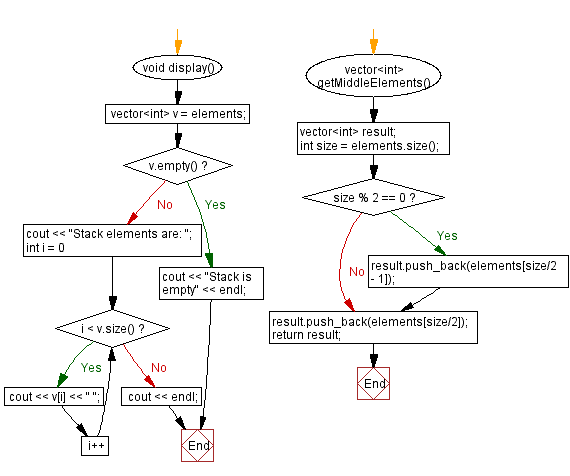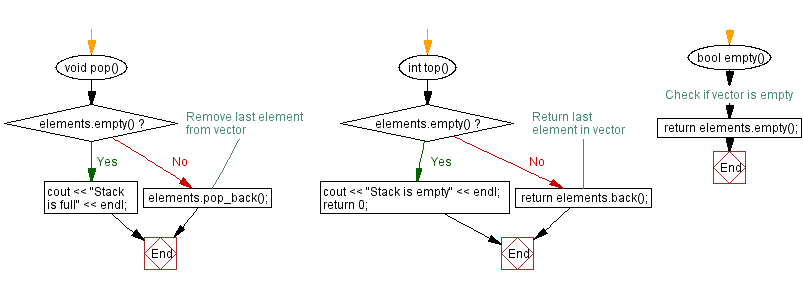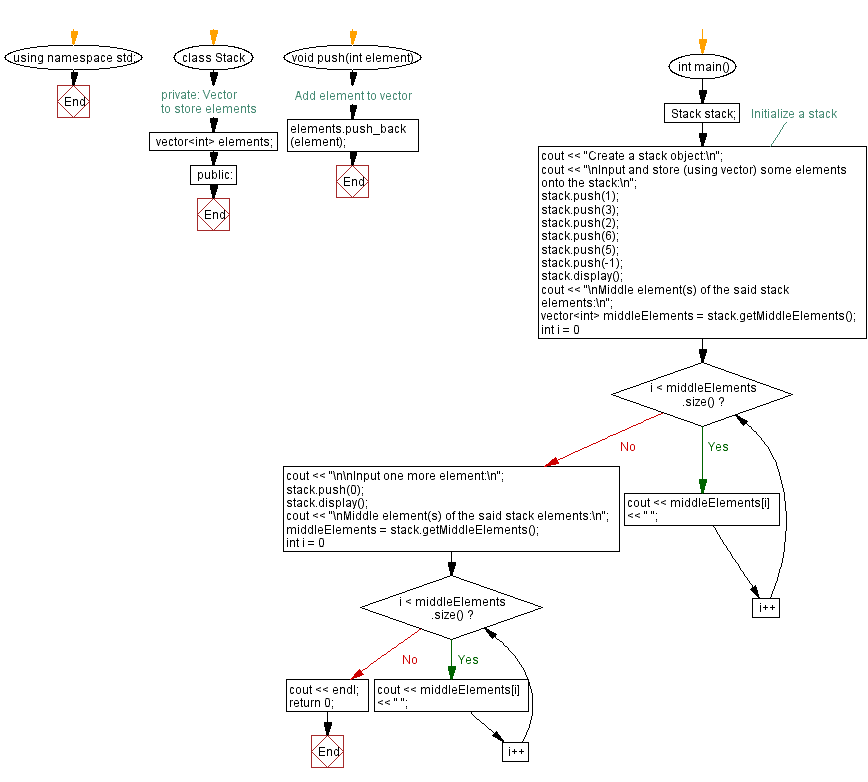﻿ C++ Find the middle element(s) of a stack (using a vector)

# C++ Stack Exercises: Find the middle element(s) of a stack (using a vector)

## C++ Stack: Exercise-27 with Solution

Write a C++ program to find the middle element(s) of a stack (using a vector).

Test Data:
Create a stack object:
Input and store (using vector) some elements onto the stack:
Stack elements are: 1 3 2 6 5 -1
Middle element(s) of the said stack elements:
2 6

Sample Solution:

C++ Code:

``````#include <iostream>
#include <vector>
#include<algorithm>

using namespace std;

class Stack {
private:
// Vector to store elements
vector<int> elements;

public:
void push(int element) {
elements.push_back(element);
}

void pop() {
if (elements.empty()) {
cout << "Stack is full" << endl;
} else {
// Remove last element from vector
elements.pop_back();
}
}

int top() {
if (elements.empty()) {
cout << "Stack is empty" << endl;
return 0;
} else {
// Return last element in vector
return elements.back();
}
}

bool empty() {
// Check if vector is empty
return elements.empty();
}

void display() {
vector<int> v = elements;
if (v.empty()) {
cout << "Stack is empty" << endl;
return;
}
cout << "Stack elements are: ";
for (int i = 0; i < v.size(); i++) {
cout << v[i] << " ";
}
cout << endl;
}

vector<int> getMiddleElements() {
vector<int> result;
int size = elements.size();
if (size % 2 == 0) {
result.push_back(elements[size/2 - 1]);
}
result.push_back(elements[size/2]);
return result;
}
};

int main() {
Stack stack;
//Initialize a stack
cout << "Create a stack object:\n";
cout << "\nInput and store (using vector) some elements onto the stack:\n";
stack.push(1);
stack.push(3);
stack.push(2);
stack.push(6);
stack.push(5);
stack.push(-1);
stack.display();
cout << "\nMiddle element(s) of the said stack elements:\n";
vector<int> middleElements = stack.getMiddleElements();
for (int i = 0; i < middleElements.size(); i++) {
cout << middleElements[i] << " ";
}
cout << "\n\nInput one more element:\n";
stack.push(0);
stack.display();
cout << "\nMiddle element(s) of the said stack elements:\n";
middleElements = stack.getMiddleElements();
for (int i = 0; i < middleElements.size(); i++) {
cout << middleElements[i] << " ";
}
cout << endl;
return 0;
}
``````

Sample Output:

```Create a stack object:

Input and store (using vector) some elements onto the stack:
Stack elements are: 1 3 2 6 5 -1

Middle element(s) of the said stack elements:
2 6

Input one more element:
Stack elements are: 1 3 2 6 5 -1 0

Middle element(s) of the said stack elements:
6
```

Flowchart:CPP Code Editor: